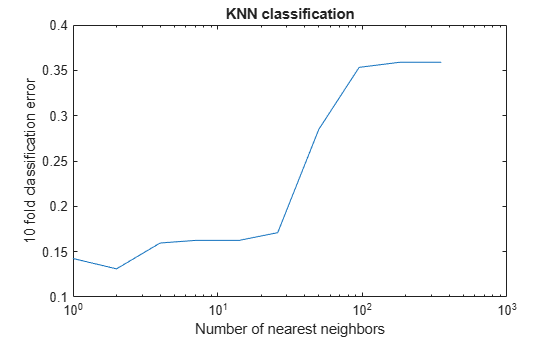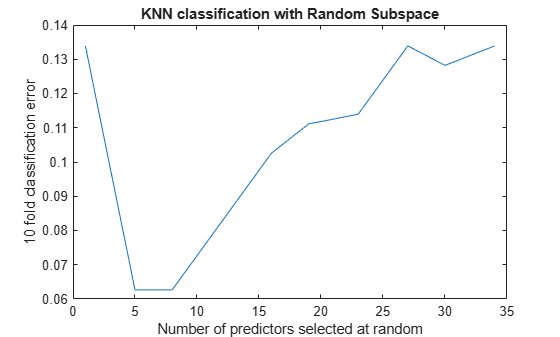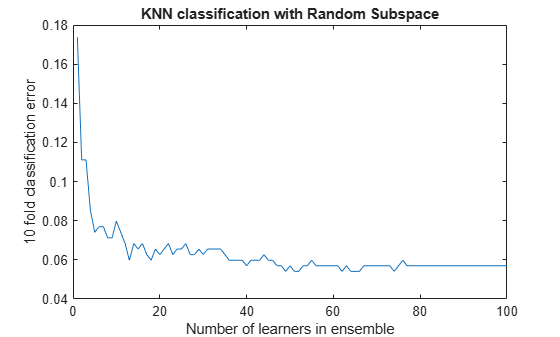# Random Subspace Classification

This example shows how to use a random subspace ensemble to increase the accuracy of classification. It also shows how to use cross validation to determine good parameters for both the weak learner template and the ensemble.

Load the `ionosphere` data. This data has 351 binary responses to 34 predictors.

```load ionosphere; [N,D] = size(X)```
```N = 351 ```
```D = 34 ```
`resp = unique(Y)`
```resp = 2x1 cell {'b'} {'g'} ```

### Choose the number of nearest neighbors

Find a good choice for `k`, the number of nearest neighbors in the classifier, by cross validation. Choose the number of neighbors approximately evenly spaced on a logarithmic scale.

```rng(8000,'twister') % for reproducibility K = round(logspace(0,log10(N),10)); % number of neighbors cvloss = zeros(numel(K),1); for k=1:numel(K) knn = fitcknn(X,Y,... 'NumNeighbors',K(k),'CrossVal','On'); cvloss(k) = kfoldLoss(knn); end figure; % Plot the accuracy versus k semilogx(K,cvloss); xlabel('Number of nearest neighbors'); ylabel('10 fold classification error'); title('k-NN classification');```The lowest cross-validation error occurs for `k = 2`.

### Create the ensembles

Create ensembles for `2`-nearest neighbor classification with various numbers of dimensions, and examine the cross-validated loss of the resulting ensembles.

This step takes a long time. To keep track of the progress, print a message as each dimension finishes.

```NPredToSample = round(linspace(1,D,10)); % linear spacing of dimensions cvloss = zeros(numel(NPredToSample),1); learner = templateKNN('NumNeighbors',2); for npred=1:numel(NPredToSample) subspace = fitcensemble(X,Y,'Method','Subspace','Learners',learner, ... 'NPredToSample',NPredToSample(npred),'CrossVal','On'); cvloss(npred) = kfoldLoss(subspace); fprintf('Random Subspace %i done.\n',npred); end```
```Random Subspace 1 done. Random Subspace 2 done. Random Subspace 3 done. Random Subspace 4 done. Random Subspace 5 done. Random Subspace 6 done. Random Subspace 7 done. Random Subspace 8 done. Random Subspace 9 done. Random Subspace 10 done. ```
```figure; % plot the accuracy versus dimension plot(NPredToSample,cvloss); xlabel('Number of predictors selected at random'); ylabel('10 fold classification error'); title('k-NN classification with Random Subspace');```The ensembles that use five and eight predictors per learner have the lowest cross-validated error. The error rate for these ensembles is about 0.06, while the other ensembles have cross-validated error rates that are approximately 0.1 or more.

### Find a good ensemble size

Find the smallest number of learners in the ensemble that still give good classification.

```ens = fitcensemble(X,Y,'Method','Subspace','Learners',learner, ... 'NPredToSample',5,'CrossVal','on'); figure; % Plot the accuracy versus number in ensemble plot(kfoldLoss(ens,'Mode','Cumulative')) xlabel('Number of learners in ensemble'); ylabel('10 fold classification error'); title('k-NN classification with Random Subspace');```There seems to be no advantage in an ensemble with more than 50 or so learners. It is possible that 25 learners gives good predictions.

### Create a final ensemble

Construct a final ensemble with 50 learners. Compact the ensemble and see if the compacted version saves an appreciable amount of memory.

```ens = fitcensemble(X,Y,'Method','Subspace','NumLearningCycles',50,... 'Learners',learner,'NPredToSample',5); cens = compact(ens); s1 = whos('ens'); s2 = whos('cens'); [s1.bytes s2.bytes] % si.bytes = size in bytes```
```ans = 1×2 1748467 1518820 ```

The compact ensemble is about 10% smaller than the full ensemble. Both give the same predictions.